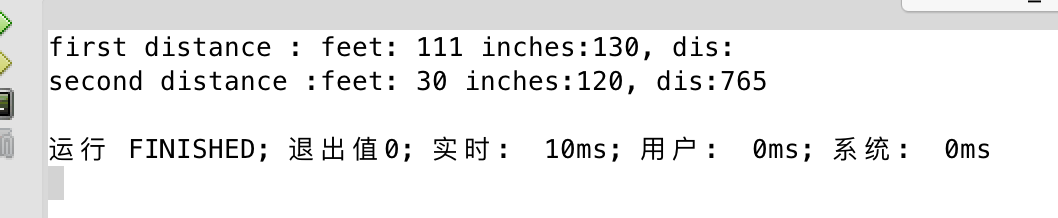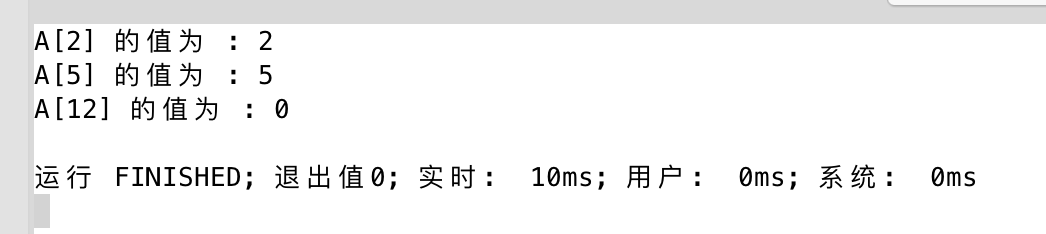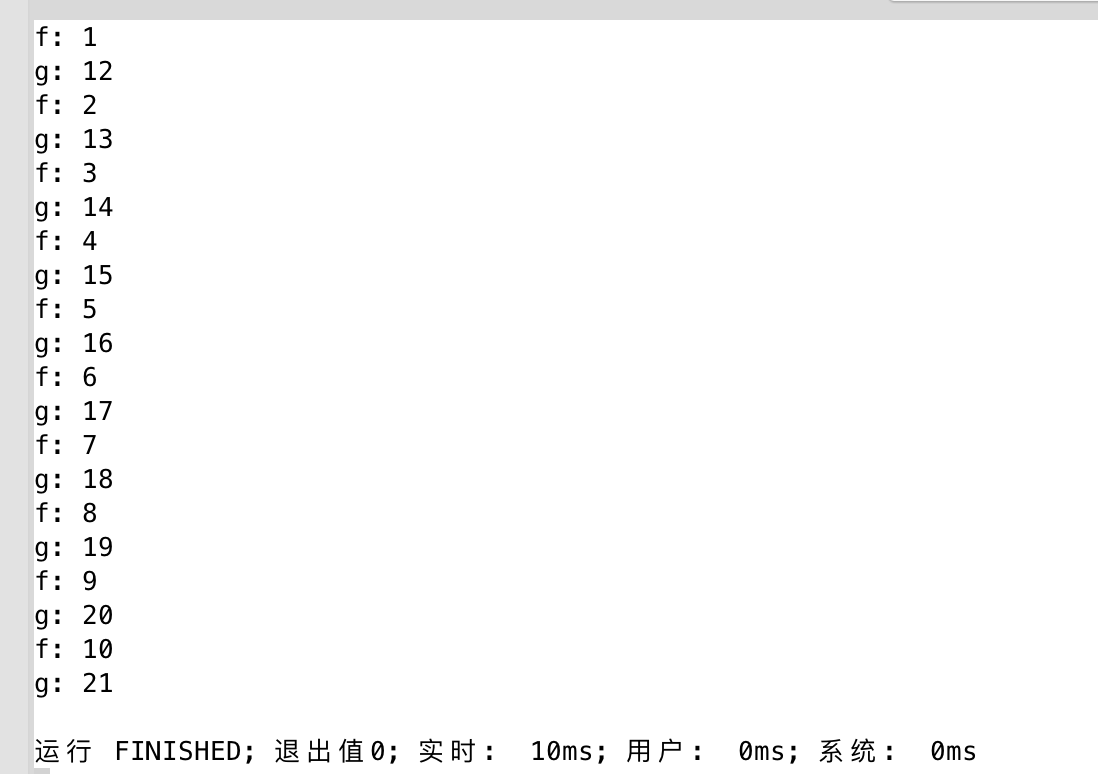# C++语言之重载运算符()/[]/++/->等运算符

### 知识点

1. 重载()运算符；
2. 重载[]运算符；
3. 重载++和->运算符；
4. 新名词记录{operator}

### 重载()运算符

class Distance
{
//私有成员
private:
int feet;
int inches;
char dis;
public:
// 所需的构造函数
Distance(){
feet = 0;
inches = 0;
}
Distance(int f, int i){
feet = f;
inches = i;
}
// 这是创建一个可以传递任意数目参数的运算符函数。
/**
* 重载了括号（），
* 传入4个参数，分别进行初始化，最后将新的对象返回出去
*/
Distance operator ()(int a, int b, int c, char d)
{
Distance D;
// 进行随机计算
D.feet = a + c + 10;
D.inches = b + c + 100 ;
strcpy(D.dis, d);
//返回新的Distance对象
return D;
}
//打印距离的方法
void displayDistance()
{
cout << "feet: " << feet <<  " inches:" <<  inches << ", dis:" << dis << endl;
}
};


void kuohaoTest(){
Distance D1(111, 130), D2;

cout << "first distance : ";
D1.displayDistance();

//调用distance变量的（）方法，需要对应参数的个数
D2 = D1(10, 10, 10, "765");
cout << "second distance :";
D2.displayDistance();
}### 重载 []

const int SIZE = 10;
class safearray
{
private:
int arr[SIZE];
public:
safearray()
{
register int i;
for(i = 0; i < SIZE; i++)
{
arr[i] = i;
}
}

int & operator[](int i)
{
if( i > SIZE )
{
//当传入的index大于数组的长度，则返回第一个元素
return arr;
}
return arr[i];
}
};


/**
* 重载方括号，保证数组不会越界
*/
void fangkuohaoTest(){
safearray A;
cout << "A 的值为 : " << A <<endl;
cout << "A 的值为 : " << A<<endl;
cout << "A 的值为 : " << A<<endl;
}### 重载++ / ->

class obj {
static int i, j;
public:
void f() const { cout << "f: " << i++ << endl; }
void g() const { cout << "g: " << j++ << endl; }
};

// 静态成员定义
int obj::i = 1;
int obj::j = 12;

// 为上面的类实现一个容器
class objContainer
{
vector<obj*> a;
public:
{
a.push_back(obj);  //调用向量的标准方法
}

friend class smartPointer;
};

// 实现智能指针，用于访问类 Obj 的成员
class smartPointer {
objContainer oc;
int index;
public:
smartPointer(objContainer &objc)
{
oc = objc;
index = 0;
}
// 返回值表示列表结束
bool operator ++ () // 前增量，只有一个括号
{
if(index >= oc.a.size()) return false;
if(oc.a[++index] == 0) return false;//index加1
return true;
}

bool operator ++ (int) // 后增量，括号里面有一个int类型
{
return operator++();
}

// 重载运算符 ->
obj* operator -> () const
{
if(!oc.a[index])
{
cout << "Zero value";
return (obj*)0;
}
//不为空，指向oc.a向量里面的obj类的实例
return oc.a[index];
}

};

void pointerTest(){
const int sz = 10;
obj o[sz];
objContainer oc;
for(int i = 0; i < sz; i++)
{
}
smartPointer sp(oc); // 创建一个迭代器

do {
sp->f(); // 智能指针调用
sp->g();
} while(sp++);
}### 总结

04-303万+
03-027万+09-072万+
09-171158
08-031万+
05-116857
07-031万+
08-27178
07-237万+